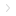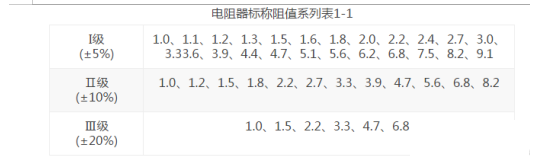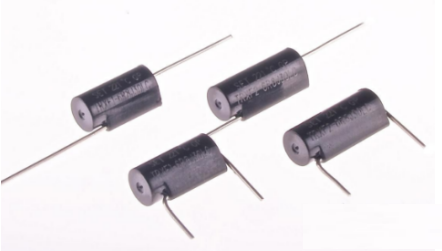Homenewsenterprise

## Understanding the Role of Resistors in Circuits

2019-05-10 07:25:12

Resistors are made by using the characteristics of some materials which hinder the current. They are the most basic and commonly used electronic components. Resistors are widely used in circuits, which can be roughly summarized as reducing voltage, distributing voltage, limiting current and providing necessary working conditions (voltage or current) for various components. In order to express conveniently, resistors are usually referred to as resistors.Understanding the role of resistors in circuits

main parameter

The main technical parameters of resistors are nominal resistance, allowable deviation and rated power.The basic unit of resistance value (referred to as resistance value) is Ohm (referred to as Ohm), which is expressed by the Greek letter "omega". Usually units larger than ohms are used - kiloohms (k_) and megaohms (M_). The conversion relationship between them is:

Understanding the role of resistors in circuits

In order to meet different needs, the state has stipulated a series of resistance values as product standards, and clearly labeled on the product standard resistance values, known as nominal resistance. The nominal resistance series of resistors in China is shown in Table 2-1. The cardinality of the ratio given in the table can be multiplied by 10.100.1000 - - - for example, 3.9_, 39_, 390_, 3.9_, 39_, 390_and 3.9 M.Understanding the role of resistors in circuits

However, due to the errors in the production process, the nominal resistance is not 100% equivalent to the actual resistance of the resistor.  The difference between the actual resistance and nominal resistance of resistors is usually expressed as the percentage of the difference and nominal resistance, which is called allowable deviation (or resistance error). Resistor products can be divided into three grades according to the allowable deviation, that is, the allowable deviation of stage is (+5%), the allowable deviation of stage II is (+10%) and the allowable deviation of stage III is (+20%). Obviously, the smaller the allowable deviation, the higher the resistance accuracy of the resistor. Resistor is a kind of energy-consuming component. When the current passes through the resistor, a part of the electric energy will be converted into heat energy, which makes the temperature of the resistor rise. If the current through the resistor is too large or the voltage at both ends of the resistor is too high, the resistor will be damaged due to overheating. Therefore, all kinds of resistors prescribe their nominal power (also known as rated power). If the power is lower than the rated power, the life of the resistor will be longer and work safely; if overloaded, the life of the lighter will be shortened, and the heavier may burn the resistor out. The maximum electrical power allowed by the resistor for long-term operation is rated power, in watts (W). General resistors are divided into 1/16W, 1/8W, 1/4W, 1/2W, 1W, 2W, 5W, 10W and so on. The actual power consumed by using medium resistors must be less than its rated power. In electronic fabrication, 1/8W or 1/4W resistors can usually be used if there is no special indication in the circuit.

Understanding the role of resistors in circuitsThe Role of Resistors in Circuits

1. Current limiting

In order to ensure the normal operation of the electrical appliances, a variable resistor can be connected in series in the circuit so that the current used in the electrical appliances does not exceed the rated value or the specified value required for the actual operation. When the resistance is changed, the magnitude of the current changes. We call this resistance, which can limit the magnitude of the current, a current limiting resistance. For example, in the circuit of adjustable lamp, in order to control the brightness of the lamp, a current limiting resistor can also be inserted into the circuit. By adjusting the size of the access resistance, the current in the circuit can be controlled to control the brightness of the lamp.

2. Shunt

When several electrical appliances with different rated currents need to be connected at the same time on the trunk of the circuit, a resistor can be connected in parallel at both ends of the appliances with lower rated currents, which functions as a "shunt". For example, there are two bulbs, A and B. The rated current is 0.2A and 0.4A, respectively. Obviously, the two bulbs can not be connected directly in series to the same circuit. But if we have a suitable shunt resistance at both ends of the lamp, when the switch S is closed, the lamp A and B can work normally.

For example, in the experimental design of resistance measurement by voltage-deficiency meter, the experimental circuit shown in Figure 3 can be designed. The shunt resistance R is connected in parallel with the resistance to be measured, the trunk current and the current in shunt resistance R are measured by ammeter, and the resistance value of the resistance to be measured can be calculated by shunt formula. If there is only one ammeter, the ammeter can be connected successively to the main road or different branches to measure I and (or sum or sum) and can also be calculated.

3. Partial pressure

Normally, the rated voltage is marked on the electrical appliances. If the power supply is higher than the rated voltage of the electrical appliances, the electrical appliances can not be directly connected to the power supply. In this case, a suitable resistance value can be connected in series to the electrical appliances to share a part of the voltage, so that the electrical appliances can work under the rated voltage. We call such resistors piezoelectric resistors.

4. Converting Electric Energy into Internal Energy

When the current passes through the resistor, all (or part) of the electric energy is converted into internal energy. The electric appliances used to convert electric energy into internal energy are called electric heaters. Such as soldering iron, electric stove, rice cooker, heater, etc.